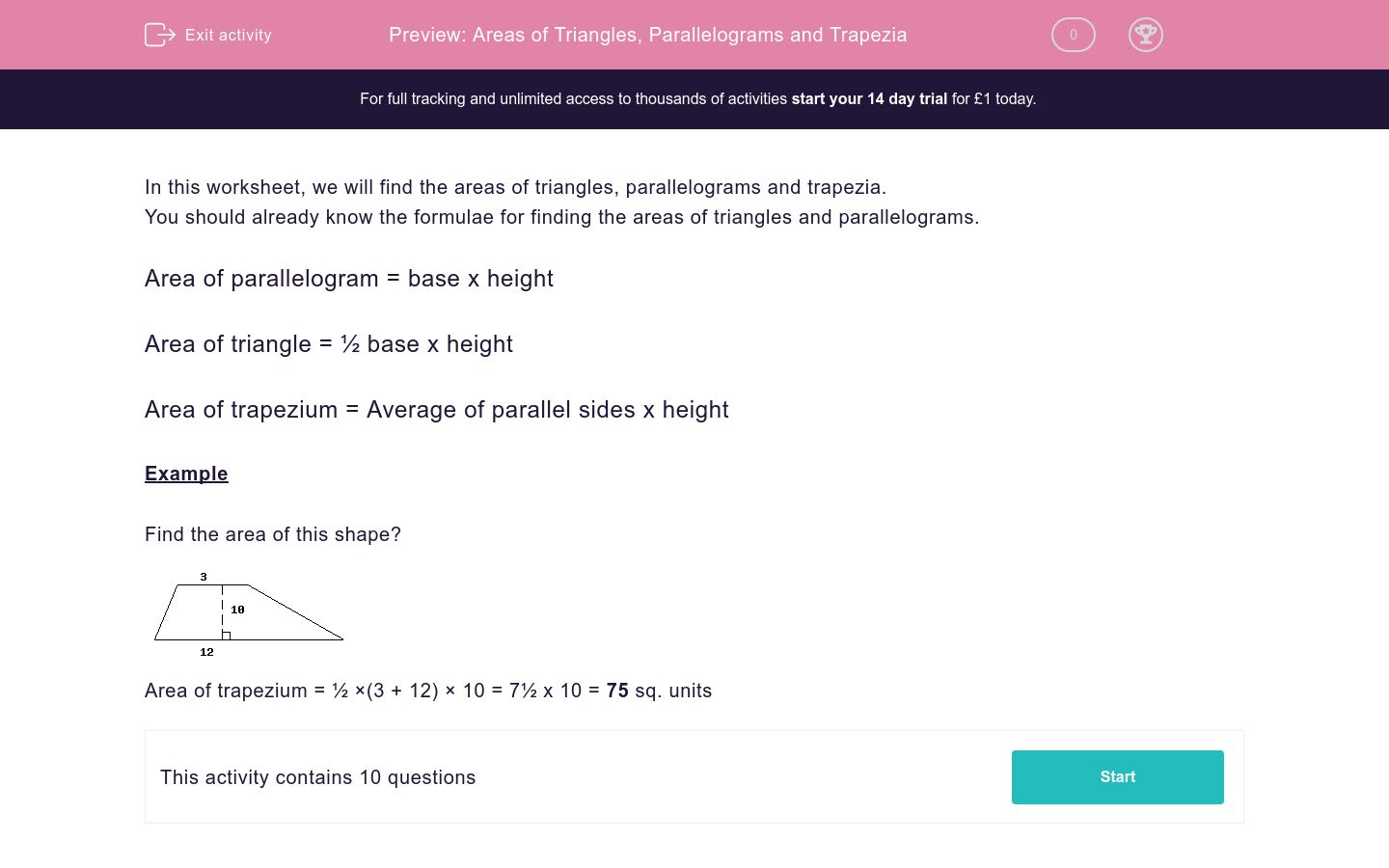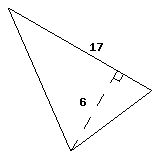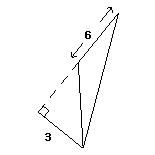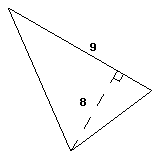# Areas of Triangles, Parallelograms and Trapezia

In this worksheet, students find the areas of triangles, parallelograms and trapezia.Key stage:  KS 2

Curriculum topic:   Maths and Numerical Reasoning

Curriculum subtopic:   2D Shapes: Circles, Angles and Bearings

Difficulty level:### QUESTION 1 of 10

In this worksheet, we will find the areas of triangles, parallelograms and trapezia.

You should already know the formulae for finding the areas of triangles and parallelograms.

Area of parallelogram = base x height

Area of triangle = ½ base x height

Area of trapezium = Average of parallel sides x height

Example

Find the area of this shape?Area of trapezium = ½ ×(3 + 12) × 10 = 7½ x 10 = 75 sq. units

Find the area of this trapezium.

(Just write the number for the whole of this worksheet)Find the area of this trapezium.Find the area of this trapezium.Find the area of this shape.Find the area of this shape.Find the area of this shape.Find the area of this trapezium.Find the area of this shape.Find the area of this shape.Find the area of this shape.• Question 1

Find the area of this trapezium.

(Just write the number for the whole of this worksheet)48
EDDIE SAYS
Area of a trapezium = 1/2 x (a + b ) x h 1/2 x (9+3) x 8
• Question 2

Find the area of this trapezium.28
EDDIE SAYS
Area of a trapezium = 1/2 x (a + b ) x h 1/2 x (6+2) x 7
• Question 3

Find the area of this trapezium.44
EDDIE SAYS
Area of a trapezium = 1/2 x (a + b ) x h 1/2 x (3+8) x 8
• Question 4

Find the area of this shape.51
EDDIE SAYS
Area of a triangle = 1/2 x b x h A = 1/2 x 6 x 17
• Question 5

Find the area of this shape.75
EDDIE SAYS
Area of a triangle = 1/2 x b x h A = 1/2 x 10 x 15
• Question 6

Find the area of this shape.98
EDDIE SAYS
Area of a parallelogram = b x h A = 14 x 7
• Question 7

Find the area of this trapezium.22
EDDIE SAYS
Area of a trapezium = 1/2 x (a + b ) x h 1/2 x (9+2) x 4
• Question 8

Find the area of this shape.25
EDDIE SAYS
Area of a triangle = 1/2 x b x h A = 1/2 x 5 x 10
• Question 9

Find the area of this shape.9
EDDIE SAYS
Area of a triangle = 1/2 x b x h A = 1/2 x 6 x 3
• Question 10

Find the area of this shape.36
EDDIE SAYS
Area of a triangle = 1/2 x b x h A = 1/2 x 9 x 8
---- OR ----